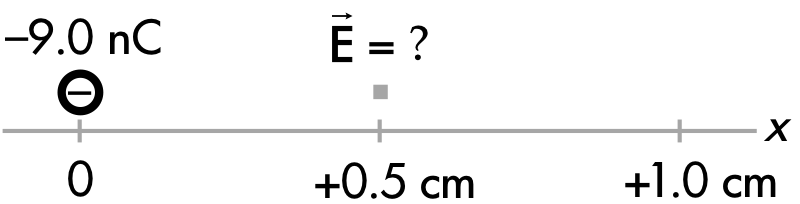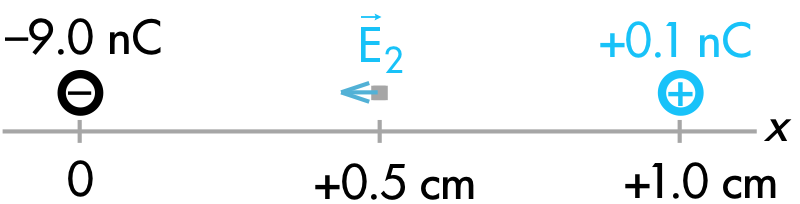## 20180307

### Physics quiz question: change(?) in magnitude of total electric field

Physics 205B Quiz 3, spring semester 2018
Cuesta College, San Luis Obispo, CAOriginally a –9.0 nC charge is held at the origin.Subsequently, if a +0.1 nC point charge were to be held in place at x = +1.0 cm, the magnitude of the electric field at x = +0.5 cm would:
(A) decrease.
(B) be unchanged.
(C) increase.
(D) (Not enough information is given.)

Correct answer (highlight to unhide): (C)The electric field vector at x = 0.5 cm due to the –9.0 nC source charge points to the left, in towards this negative source charge.The electric field vector at x = 0.5 cm due to the +0.1 nC source charge also points to the left, out away from this positive source charge.The total electric field at x = 0.5 cm due to both source charges is the vector addition of the individual electric field vectors at that location. Since the electric fields due to the –9.0 nC source charge and the +0.1 nC source charge point in the same directions at x = 0.5 cm, the direction of the total electric field there would point to the left, and its magnitude would be greater than just the electric field of the –9.0 nC source charge alone).

Sections 30882, 30883
Exam code: quiz03Ch4R
(A) : 10 students
(B) : 8 students
(C) : 14 students
(D) : 0 students

Success level: 44% (including partial credit for multiple-choice)
Discrimination index (Aubrecht & Aubrecht, 1983): 0.64